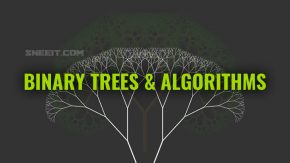# Binary Search

Using splitting method to search a value in an ordered collection or array, Binary Search is a the most fundamental and useful algorithmsUsing splitting method to search a value in an ordered collection or array, Binary Search is a the most fundamental and useful algorithms in Computer Science.

## Background

To find a target in an ordered collection, we can split that collection into two search spaces indicated by start, mid, end indices and compare the target with the mid element. If target = mid element => return. If target < mid, keep the start-mid space for the next split, otherwise, if target > mid, keep the mid-right space for the next split. Operating the splits until target = mid or our search spaces are empty.

If a problem asks for finding / searching something in a sorted / partly-sorted collection within O(log n) time complexity, that’s a sense of using Binary Search algorithm. You can try to solve Binary Search problem to become familiar with the code structure of this kind of problem.

## Template

Here is the code for the Binary Search problem problem:

```var search = function(nums, target) {
// pre-processing, ex: sort array if need
// ...

// initialize search space
let start = 0, end = nums.length - 1, mid = null;

// termination condition
while (start < end) {
// in-loop-processing
// ...

// calculate mid
mid = start + Math.floor((end - start) / 2);

// select search space
if (target > nums[mid]) {
// exclude mid because target is
// impossible in mid or above
start = mid + 1;
} else {
end = mid
}
}

// start == end
// post-processing
return nums[start] == target ? start : -1
};```

There are 5 main parts in a code for every binary search problems that you can customize:

1. initialize search space: must set start and end to form a search space which covers ALL cases / candidates / possible elements
2. termination condition: I like to use start < end then at the end: start == end so I don’t have to think about the what variable will be used for my post-processing.
3. calculate mid: I use mid = start + Math.floor((end  – start) / 2) to avoid overflow and mid is always smaller than right so we will never get infinite loop in case the search space has only 2 or lower number of elements.
4. select search space: reduce our search space to the space that includes the target. I usually use start = mid + 1 (if target > mid), OTHERWISE, start = mid.
5. post-processing: we process our start to return the result as the requirements of the problem

## Applications

If you notice, there is no condition target == nums[mid] in my loop, so even target == nums[mid], the loop will continue until it find:

• start = lowest index that nums[index] = target if mid calculation is: mid = start + Math.floor((end – end) / 2). I call this is “focus start” template. This is very useful to solve the problems related to counting like: Kth Smallest Element in a Sorted Matrix
• start = highest index that nums[index] = target if mid calculation is: mid = start + Math.floor((end + 1 – start) / 2). I call it “focus end“.  If you use this, please make sure you change the search space selection to: start= mid + 1 (if target > the mid) AND end = mid (if target <= the mid).

*Note: You may need to add target == nums[mid] in your loop in some cases (like there are too many repeating values), so just analyze your problem and make rational modifications to the template.

In the case when the target is not in our nums:

• the “focus start” template return start right above the target: nums[start-1] < target < nums[start]
• the “focus end” template return start right below the target: nums[start] < target < nums[start+1]

## Practices